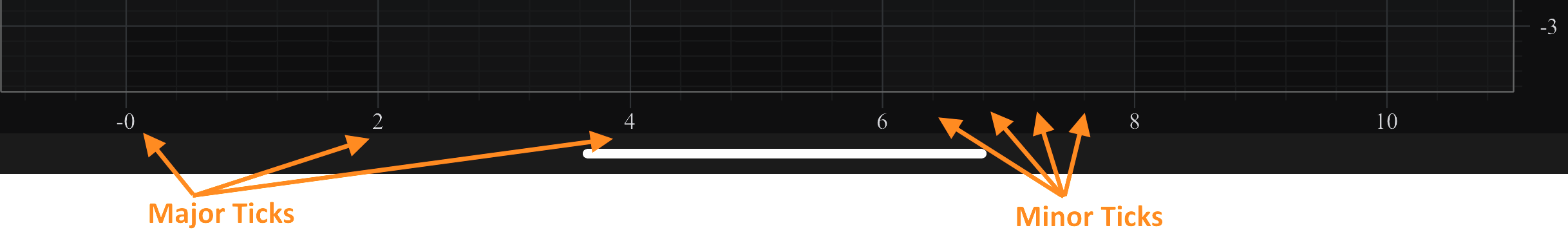iOS & macOS Charting Documentation - SciChart iOS & macOS Charts SDK v4.x

# Axis Ticks - MajorDelta, MinorDelta and AutoTicks

## Axis Ticks, Labels and Grid Lines

In SciChart, the Ticks are small marks around the chart on an axis. There are Minor and Major Ticks, where Minor Ticks are placed in between Major ones. By default, Major Ticks are longer and thicker than Minor Ticks.

Axis Labels appears for every Major Tick, if there is enough space around. If Axis Labels are placed too tightly, some of them get culled to make more space. If necessary, labels culling can be switched off via `ISCIAxis.isLabelCullingEnabled` property.

Grid Lines correspond to Ticks on an axis. Likewise, there are Minor and Major Grid lines. In SciChart, axes are responsible not only for drawing Ticks and Labels, but also for the chart grid. An axis draws only those Grid Lines that can be measured against it, i.e. a horizontal axis draws vertical grid lines and vice versa.majorDelta = 2; minorDelta = 0.4; autoTicks = NO

To learn more about possible options for Axis Ticks, Gridlines and Labels, please refer to the Styling Gridlines, Ticks and Axis Bands and Styling Title and Axis Labels articles.

## Automatic Tick Spacing

In SciChart, the difference between two Major Ticks is called MajorDelta, and that between two minor Ticks - MinorDelta. By default, both delta values, and therefore the Tick spacing, are calculated automatically according to the VisibleRange and size of an axis. As zoom level changes, the `ISCIAxisCore.majorDelta`, `ISCIAxisCore.minorDelta` of every axis will be updated correspondingly, and the tick frequency may change.

There are two additional options used to control the auto ticking behavior. The `ISCIAxisCore.maxAutoTicks` property allows to limit the number of generated ticks to the desired number, and the `ISCIAxisCore.minorsPerMajor` - specifies how many Minor Ticks are drawn between two Major Ticks (the default is 5).

id<ISCIAxis> axis = [SCINumericAxis new]; // change max possible auto ticks amount (the default is 10) axis.maxAutoTicks = 20; // specify that there should be 10 Minor Ticks between two Major Ticks (the default is 5) axis.minorsPerMajor = 10;
let axis = SCINumericAxis() // change max possible auto ticks amount (the default is 10) axis.maxAutoTicks = 20 // specify that there should be 10 Minor Ticks between two Major Ticks (the default is 5) axis.minorsPerMajor = 10
var axis = new SCINumericAxis() // change max possible auto ticks amount (the default is 10) axis.MaxAutoTicks = 20; // specify that there should be 10 Minor Ticks between two Major Ticks (the default is 5) axis.MinorsPerMajor = 10;

## Altering Tick Spacing

It is also possible to set MinorDelta and MajorDelta manually. To change them, automatic calculation must be switched off via the `ISCIAxisCore.autoTicks` property. To set delta values, call the `ISCIAxisCore.minorDelta` and `ISCIAxisCore.majorDelta` properties. Please note that both delta values need to be set in this case:

id<ISCIAxis> axis = [SCINumericAxis new]; axis.autoTicks = NO; axis.minorDelta = @(2); axis.majorDelta = @(10);
let axis = SCINumericAxis() axis.autoTicks = false axis.minorDelta = NSNumber(value: 2) axis.majorDelta = NSNumber(value: 10)
var axis = new SCINumericAxis() axis.AutoTicks = false; axis.MinorDelta = 2.FromComparable(); axis.MajorDelta = 10.FromComparable();

For `SCIDateAxis` the majorDelta and minorDelta should be set with a Date object whose tick value is the desired timespan.

## Altering Tick Spacing for the Logarithmic Axis

`SCILogarithmicNumericAxis` is a special case that need to be mentioning here. Due to the exponential nature of this axis type, MajorDelta represents the difference between exponents of neighbouring major ticks, not between their actual values. For instance, having `ISCIAxisCore.majorDelta` = 3 and `ISCILogarithmicNumericAxis.logarithmicBase` = 10 on a `SCILogarithmicNumericAxis` specifies that major ticks and gridlines will be spaced at 103 intervals (exponents will be divisors of MajorDelta):LogarithmicAxis, MajorDelta = 3, minor ticks/gridlines hidden

On the contrary, the difference between neighbouring minor ticks is not exponential. Therefore, MinorDelta represents the difference between the actual values of neighbouring minor ticks.

For instance, having `ISCIAxisCore.minorDelta` = 100 results in minor ticks and gridlines appearing with the increment of 100x10E, where `E` is the exponent of the nearest major tick to the left. So, the interval will be like the following:

Range Interval Raw Interval
[1x100, 1x103] 100x100 100
[1x103, 1x106] 100x103 100000
[1x106, 1x109] 100x106 100000000LogarithmicAxis, MajorDelta = 3, MinorDelta = 100